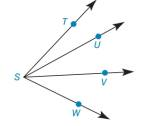Chapter 1.4, Problem 43E### Elementary Geometry for College St...

6th Edition
Daniel C. Alexander + 1 other
ISBN: 9781285195698

#### Solutions

Chapter
Section### Elementary Geometry for College St...

6th Edition
Daniel C. Alexander + 1 other
ISBN: 9781285195698
Textbook Problem
1 views

#Exercises 44, 45If m ∠ T S U = x + 2 z , m ∠ U S V = x − z , and m ∠ V S W = 2 x − z , find x if m ∠ T S W = 60 . Also, find z if m ∠ U S W = 3 x − 6 .

To determine

To find:

The value of x and z.

Explanation

Given:

mTSU=x+2z, mUSV=xz, mVSW=2xz, mTSW=60 and mUSW=3x6.

The given figure is,

Definition:

If a point say M lies in the interior of PQR, then mPQM+mRQM=mPQR.

Approach:

The given figure is,

Figure (1)

Consider the figure (1),

U and V are the interior point of the angle TSW.

mTSU is x+2z, mUSV is xz, mVSW is 2xz and mTSW is 60°.

Use above mentioned angle addition postulate to solve for x.

### Still sussing out bartleby?

Check out a sample textbook solution.

See a sample solution

#### The Solution to Your Study Problems

Bartleby provides explanations to thousands of textbook problems written by our experts, many with advanced degrees!

Get Started

#### Simplify the expressions in Exercises 97106. 21/32122/321/3

Finite Mathematics and Applied Calculus (MindTap Course List)

#### Calculate y'. 11. y=xcosx

Single Variable Calculus: Early Transcendentals

#### By definition the improper integral It is not an improper integral.

Study Guide for Stewart's Single Variable Calculus: Early Transcendentals, 8th

#### Graph each ellipse. x236+y225=1

College Algebra (MindTap Course List)